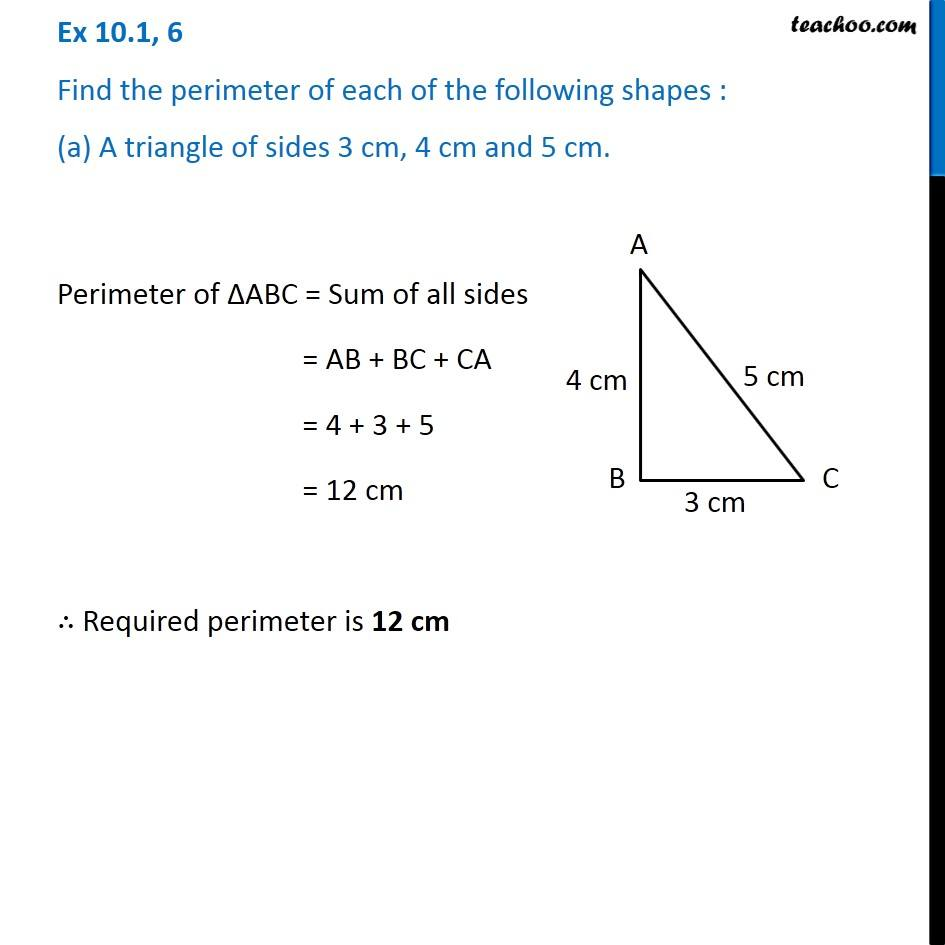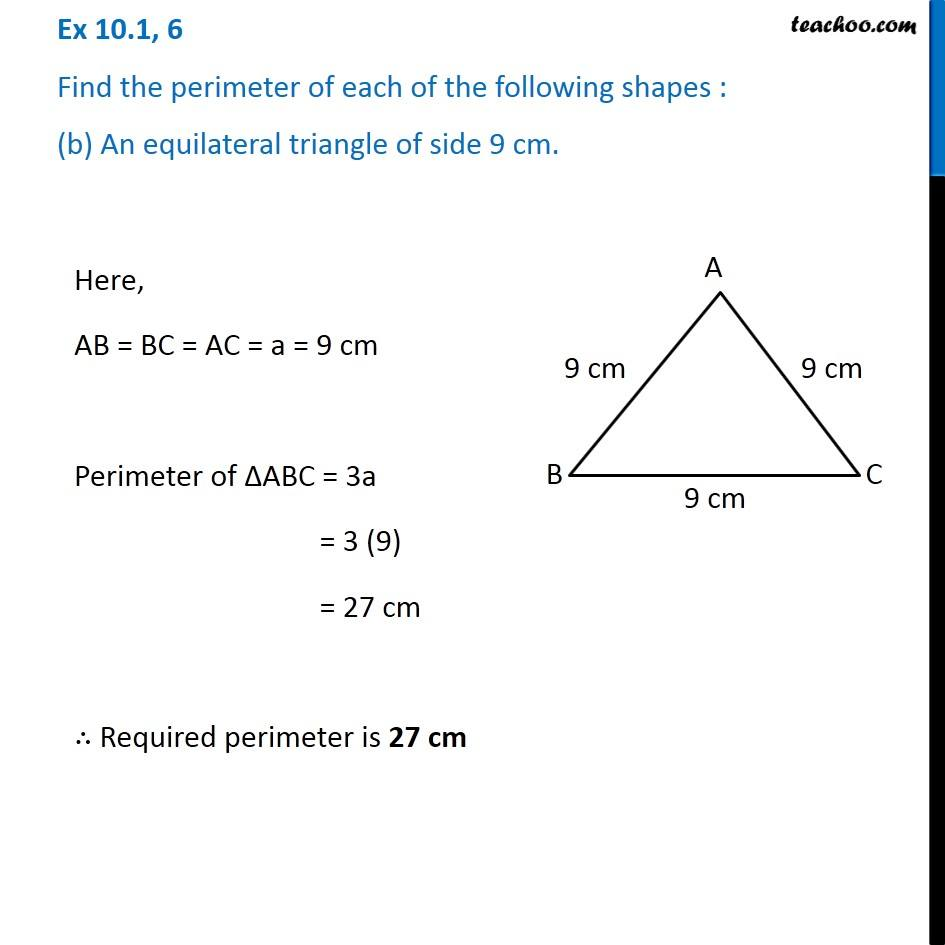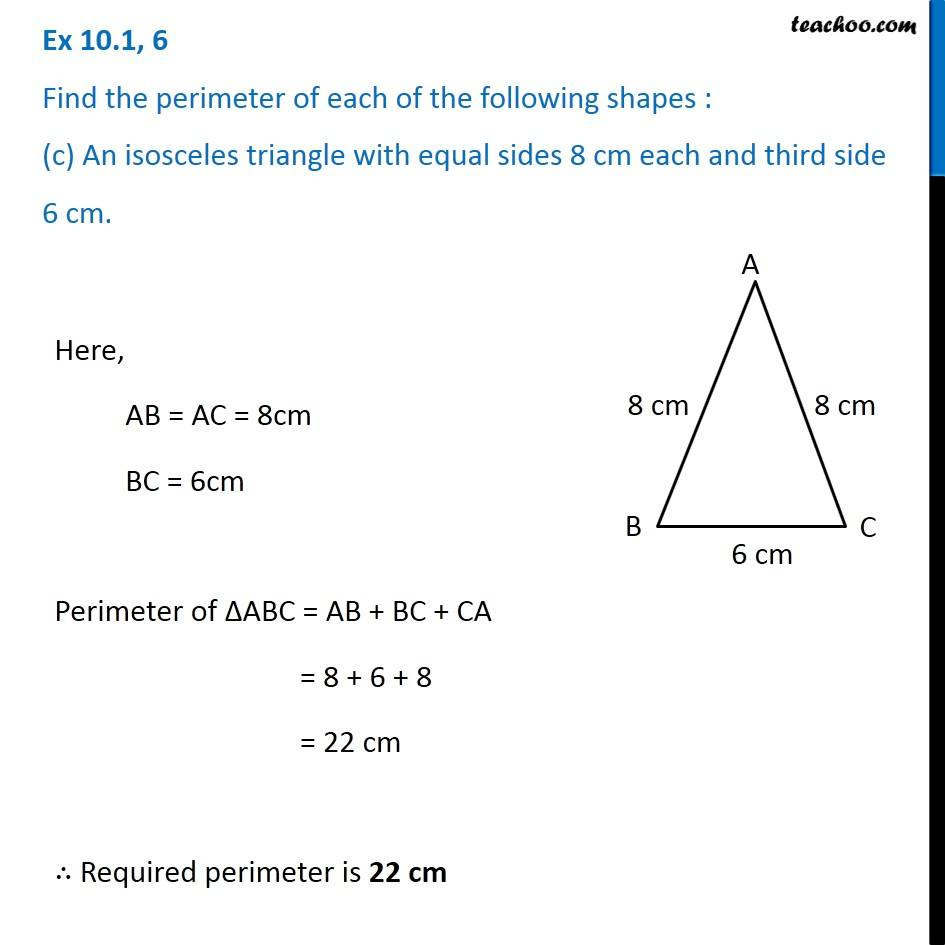Subscribe to our Youtube Channel - https://you.tube/teachoo

1. Chapter 10 Class 6 Mensuration
2. Concept wise
3. Perimeter of Triangle

Transcript

Ex 10.1, 6 Find the perimeter of each of the following shapes : (a) A triangle of sides 3 cm, 4 cm and 5 cm. Perimeter of ∆ABC = Sum of all sides = AB + BC + CA = 4 + 3 + 5 = 12 cm ∴ Required perimeter is 12 cm Ex 10.1, 6 Find the perimeter of each of the following shapes : (b) An equilateral triangle of side 9 cm. Here, AB = BC = AC = a = 9 cm Perimeter of ∆ABC = 3a = 3 (9) = 27 cm ∴ Required perimeter is 27 cm Ex 10.1, 6 Find the perimeter of each of the following shapes : (c) An isosceles triangle with equal sides 8 cm each and third side 6 cm. Here, AB = AC = 8cm BC = 6cm Perimeter of ∆ABC = AB + BC + CA = 8 + 6 + 8 = 22 cm ∴ Required perimeter is 22 cm

Perimeter of Triangle